The Lorenz model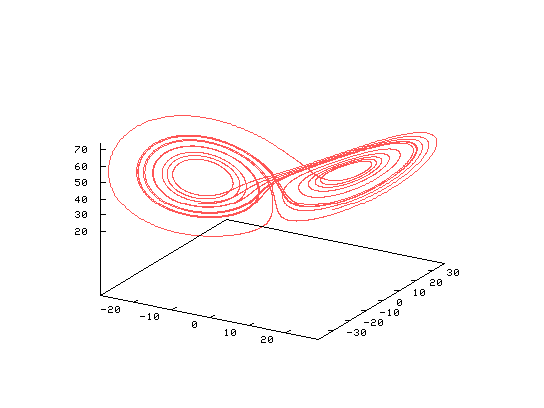If we look at the Lorenz system of equations,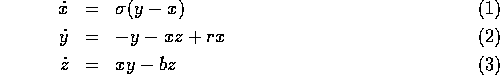The parameter r is the ratio of the Rayleigh number divided by the critical Rayleigh number. The parameter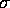is the Prandtl number. The third parameter b is related to the horizontal wave number of the system. Typical values, r = 28,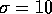,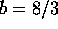, dimension. A common second set of values,,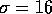, b = 4, dimension.

The interesting cases are where the Rayleigh number ratio, r, is large which suggests that we could expand the system of equations around a parameter which is proportional to the reciprocal of r (which would be small).

If we define,and let,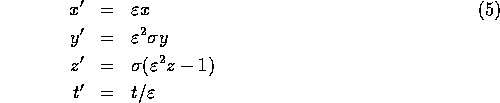Then equations (1) - (3) become (after dropping the primes),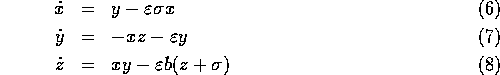Now consider the expansion of x, y, and z in terms of the parameter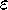,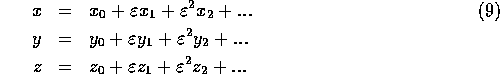Introducing (9) in (6) - (8), gives the order 0 equations,and at order, the system,The interdependence of the first order equations with the order 0 equations can removed with some algebraic manipulation,

Use (11) and (12) to eliminate,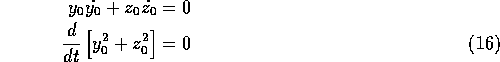Integrating this,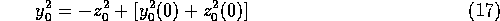where the terms in the brackets of equation (17) are the initial values of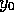and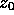. We will define this (constant) term as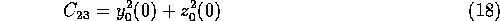If we go back to (10) and (12) to eliminate,Integrating this gives,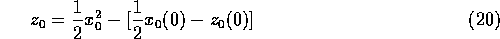here the terms in the brackets of equation (20) are the initial values ofand.

We will define this term as,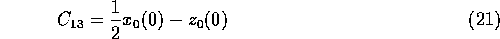Using (17) in (10) gives,now using (19),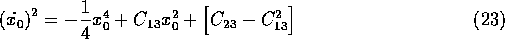Given the solution to this equation,can be solved for by using (10). Then given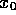and, thencan be solved for by using (12). An equation forcan also be derived by using manipulations similar to that used in deriving (23) (using equations (17) and (20) in (12) to eliminateand), to give,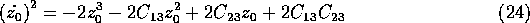Equation (23) can be solved analytically, its solution is a Jacobi elliptic function,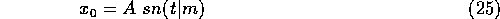The other components can also be determined,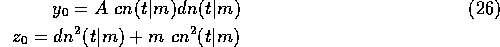(where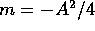) so the system is well behaved (not chaotic).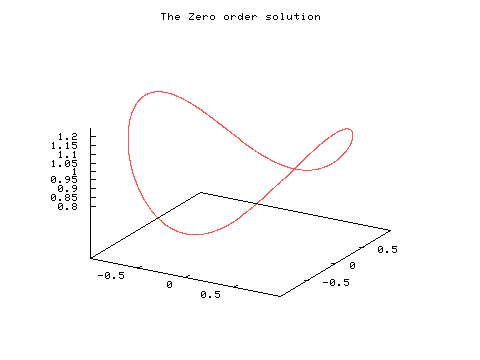The first order equations ( (13) - (15) ) are linear so they cannot possibly lead to chaotic solutions (all subsequent order equations are also linear). Thus we have shown that while the actual system can be chaotic, the perturbation solutions may not be.The above figure shows a phase portrait of the solution of the full system ( (6) - (8) in blue ), the zero order system ( (10) - (12), in red ), and the perturbation solution to first order ( i.e. withtimes the solution of (13) - (15) added to the zero order solution, in green). The perturbation solution tracks the nonlinear solution for a short while then it moves off in a different direction. The perturbation solution also rapidly grows to order one, so that the expansion (9) is valid for only a limited time.